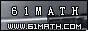Today is . WELCOME: huohai3983/44663  Home | Reg Login | All user | setHomePage | BookmarkHome | Math Test Online | Addition | Subtraction | Multiplication | Division | Time | Worksheet(JPG/PDF) | CAI | Flash Games |Recommendation articleArticle searchHome--maths--Third Grade--Estimating a sum by rounding

Estimating a sum by rounding

Estimating a sum by rounding

A quick way to estimate the sum of two numbers is to round each number and then add the rounded numbers. It is likely that this won't be the exact answer but it may be close enough for some purposes.

How to Estimate a sum by rounding.

• Round each term that will be added

Some uses of rounding are:

• Checking to see if you have about enough money to buy what you want.
• Getting a rough idea of the correct answer to a problem.

#### Estimate the Sum to the nearest thousand.

 +

2006-10-04 13:23:49
Viewed 2917 times CloseGreatmathsites